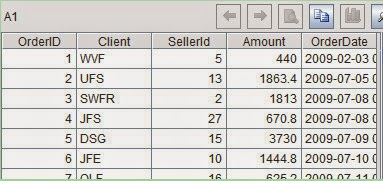## July 16, 2014

### A Code Example for Reading and Writing EXCEL Files with esProc

It is convenient to read and write Excel files with esProc. We’ll illustrate this through an example:
Example description: Read data of orders and sellers’ information list from data.xlsx, compute sales amount of every department according to the two sheets, and enter the result into result.xlsx.
Original Excel File: data.xlsx contains two sheets. The data of orders is shown in the following figure:Sellers'information list is as follows:A1-A2：Read the first sheet, data of orders, and the second sheet, sellers’ information list, respectively from the excel file, data.xlsx, and stores them in cell A1 and A2 in the form of table sequence.
A3-A4：First, make a left join of data of orders and seller’s information list according to employee ID numbers, then seek the sales amount of each department by grouping and summarizing. We won’t discuss the algorithm here for it is not important in this example.
A5：Store the result in result.xlsx.

result.xlsx

• Column name
importxls uses function option @t, which shows that the first row of sheet is regarded as column name of esProc table sequence. For example, click cell A1 and the values of its variables can be seen:Therefore, the algorithm that follows can access data in A1 using column names directly.

• Sheet name
The above code reads data according to the sheet’s serial number, but sometimes, clients prefers sheet name. This demand can be realized in esProc. For instance, “sales” is the sheet name for data of orders. In this case, reading by names will be realized only by changing the sheet’s serial number in A1 into sheet name directly. See below:
file("E:/data.xlsx").importxls@t(;”sales”)

The same will do when writing a result into a file. Say, we want to export result in A4 to a sheet named summary, the code is:
file("E:/result.xlsx").exportxls@t(A4;”summary”)

• Scope of data
The above code is to read data from the first row of sheet to the end by default. In reality, however, we are often confronted with cases that a portion of the data is to be read. As shown in the following figure:Importxls function defines the scope of row numbers waiting to be read. Say, reading from the 4th row, which could be written as:
file("E:/data.xlsx").importxls@t(;1,4)
Reading from the 4th row to 1000th row, which could be written as:
file("E:/data.xlsx").importxls@t(;1,4:1000)
Column numbers for reading can also be defined. Say, three columns OrderID, SellerId and Amount are to be read, the code for this could be:
file("E:/data.xlsx").importxls@t(OrderID,SellerId,Amount;1)